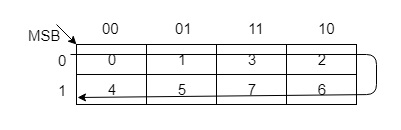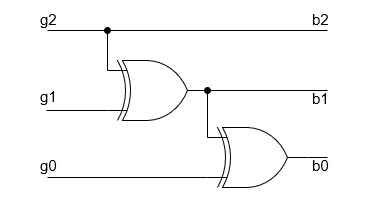# Conversion of Gray Code to Binaryn

The reflected binary code or Gray code is an ordering of the binary numeral system such that two successive values differ in only one bit (binary digit). Gray codes are very useful in the normal sequence of binary numbers generated by the hardware that may cause an error or ambiguity during the transition from one number to the next. So, the Gray code can eliminate this problem easily since only one bit changes its value during any transition between two numbers.

## Conversion of Gray to Binary Code

Gray codes are used in rotary and optical encoders, Karnaugh maps, and error detection. The hamming distance of two neighbours Gray codes is always 1 and also first Gray code and last Gray code also has Hamming distance is always 1, so it is also called Cyclic codes. You can convert a Gray code to Binary number using two methods.

Using Karnaugh (K) - map

You can construct Gray codes using other methods but they may not be performed in parallel like given above method. For example, 3 bit Gray codes can be contracted using K-map which is given as following below:Decimal
Gray Code
Binary
0
000
000
1
001
001
2
011
010
3
010
011
4
110
100
5
111
101
6
101
110
7
100
111

Using Exclusive-Or () operation

This is very simple method to get Binary number from Gray code. These are following steps for n-bit binary numbers −

• The Most Significant Bit (MSB) of the binary code is always equal to the MSB of the given binary number.
• Other bits of the output binary code can be obtained by checking gray code bit at that index. If current gray code bit is 0, then copy previous binary code bit, else copy invert of previous binary code bit.

For example, for 3-bit binary number, let Binary digits are b2 , b1 , b0, where b2 is the most significant bit (MSB) and b0 is the least significant bit (LSB) of Binary. Gray code digits are g2 , g1 , g0, where g2 is the most significant bit (MSB) and g0 is the least significant bit (LSB) of Gray code.

Gray Code
g2g1g0
Binary
b2b1b0
000
000
001
001
011
010
010
011
110
100
111
101
101
110
100
111

Therefore, you solve boolean expression using k-map, you will get b2=g2, b1=g1⊕g2, and b0=g0⊕g1⊕g2.Similarly, you can convert n-bit (bnb(n-1)...b2b1b0) Binary number into Gray code (gng(n-1)...g2g1g0). For least significant bit (LSB) bn=gn, b(n-1)=g(n-1)⊕gn, …. b1=g1⊕g2⊕g3...⊕gn and b0=g0⊕g1⊕g2⊕g3...⊕gn.

Example − Convert Gray code 100111 into Binary number.

So, according above algorithm,

b5=g5=1=1

b4=g5⊕g4 =1⊕0 =1

b3=b4⊕g3 =1⊕0 =1

b2=b3⊕g2 =1⊕1 =0

b1=b2⊕g1 =0⊕1 =1

b0=b1⊕g0 =1⊕1 =0

So, Binary number will be 111010.